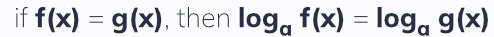top of page

#### Notes by Category University Engineering

Rate these notesNot a fanNot so goodGoodVery goodBrillRate these notes

# Exponentials and Logarithms

An exponential function is one a constant is raised to the power of a variable:• The larger the coefficient, the steeper the graph

• All exponential functions in the form y = a^x pass through (0, 1)

• The value of the function decreases as x tends to 0

Functions in the form y = a^x where 0 < a < 1 are the other way around:The gradient graphs of an exponential function are always very similar, but when a = 2.72 (e), the gradient graph is exactly the same:For all real values of x:

if f(x) = e^x, f'(x) = e^x
if f(x) = e^kx, f'(x) = ke^kx

Functions in e can be used to model growth or decline where the rate of increase in number is proportional to the number.

## Logarithms

Logarithms are the inverse of exponential functions.Just as indices laws apply to exponentials, there are a series of laws that apply to logarithms - these are known as the log rules or laws of logarithms:When working with difficult equations with exponents, it can be helpful to apply logarithms to both sides:### The Natural Logarithm, ln

The natural logarithm, ln, is the logarithm in base e. The graph of ln(x) is reflection of e^x in the line y=x:The y=axis is an asymptote in this graph, meaning that

ln(x) is only defined when x > 0

### Logarithms and Non-Linear Data

Often, it is helpful to model non-linear data as linear. This can be achieved by taking logs of one or both sides of the equation:• If the equation is in the form y = ax^n, a graph of log y against log x will give a straight line where log a is the y intercept and n the gradient.• If the equation is in the form y = ab^x, a graph of log y against x will give a straight line where log a is the y intercept and log b the gradient.

See All
bottom of page